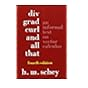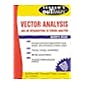Normal view

# Div, grad, curl, and all that : an informal text on vector calculus / H.M. Schey.

Material type:TextLanguage: English Publisher: New York : W.W. Norton, c2005Edition: 4th edDescription: viii, 163 p. : ill. ; 24 cmISBN: 0393925161 (pbk.); 9780393925166DDC classification: 515.63 LOC classification: QA433 | .S28 2005Online resources: WorldCat details | E-book Fulltext
Contents:
Table of contents I. Introduction, vector functions, and electrostatics -- Introduction -- Vector functions -- Electrostatics -- Problems -- II. Surface integrals and the divergence -- Gauss' law -- The unit normal vector -- Definition of surface integrals -- Evaluating surface integrals -- Flux -- Using Gauss' law to find the field -- The divergence -- The divergence in cylindrical and spherical coordinates -- The Del notation -- The divergence theorem -- Two simple applications of the divergence theorem -- Problems -- III. Line integrals and the curl -- Work and line integrals -- Line integrals involving vector functions -- Path independence -- The curl -- The curl in cylindrical and spherical coordinates -- The meaning of the curl -- Differential form of the circulation law -- Stokes' theorem -- An application of Stokes' theorem -- Stokes' theorem and simpl connected regions -- Path independence and the curl -- Problems -- IV. The gradient -- Line integrals and the gradient -- Finding the electrostatic field -- Using Laplace's equation -- Directional derivatives and the gradient -- Geometric significance of the gradient -- The gradient in cylindrical and spherical coordinates -- Problems -- Solutions to problems.
Tags from this library: No tags from this library for this title.Average rating: 0.0 (0 votes)
Item type Current location Collection Call number Copy number Status Date due Barcode Item holdsE-Book
E-book
Non-fiction 515.63 SCD 2005 (Browse shelf) Not for loanText
Reserve Section
Non-fiction 515.63 SCD 2005 (Browse shelf) C-1 Not For Loan 18922Text
515.63 SCD (Browse shelf) C-2 Available 5299-18923
Total holds: 0
##### Browsing EWU Library shelves, Shelving location: Reserve Section Close shelf browserNo cover image availableNo cover image available No cover image available 515.625 RAI 1995 Introduction to partial differential equations / 515.625 RAI 1995 Introduction to partial differential equations / 515.63 SAV A Text book on vector Analysis / 515.63 SCD 2005 Div, grad, curl, and all that : 515.63 SPS 1974 Theory and problems of vector analysis and an introduction to tensor analysis : 515.63 SPT 1995 Tensor calculus / 515.63 SPT 1995 Tensor calculus /

Includes index.

Introduction --
Vector functions --
Electrostatics --
Problems --
II. Surface integrals and the divergence --
Gauss' law --
The unit normal vector --
Definition of surface integrals --
Evaluating surface integrals --
Flux --
Using Gauss' law to find the field --
The divergence --
The divergence in cylindrical and spherical coordinates --
The Del notation --
The divergence theorem --
Two simple applications of the divergence theorem --
Problems --
III. Line integrals and the curl --
Work and line integrals --
Line integrals involving vector functions --
Path independence --
The curl --
The curl in cylindrical and spherical coordinates --
The meaning of the curl --
Differential form of the circulation law --
Stokes' theorem --
An application of Stokes' theorem --
Stokes' theorem and simpl connected regions --
Path independence and the curl --
Problems --
Line integrals and the gradient --
Finding the electrostatic field --
Using Laplace's equation --
Directional derivatives and the gradient --
Geometric significance of the gradient --
The gradient in cylindrical and spherical coordinates --
Problems --
Solutions to problems.

Applied Physics & Electronics

There are no comments for this item.